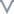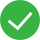# 性能测试

EBS性能测试，推荐使用fio，在centos下，通过以下命令进行安装

``````sudo yum install libaio -y
sudo yum install libaio-devel -y
sudo yum install fio -y
``````

``````fio -filename=/dev/vdb -direct=1 -iodepth=32 -thread -rw=write -ioengine=libaio -bs=1m -size=200g -numjobs=1 -runtime=300 -group_reporting -name=test
``````

-filename=/dev/vdb filename支持文件、裸盘、RBD image，这里是裸盘；如果要测文件系统的话，filename=<具体的文件名>；如果是RBD image，filename=<具体的image name> 该参数可以同时制定多个设备或文件，格式为：-filename=/dev/vdb:/dev/vdc:/dev/vdd（以冒号分割）
-direct=1 direct即使用直接写入，绕过操作系统的page cache
-iodepth=32 iodepth是设置IO队列深度，即单线程中一次给系统多少IO请求。如果使用同步方式，单线程中iodepth总是1；如果是异步方式，就可以提高iodepth，一次提交一批IO，使得底层IO调度算法可以进行合并操作。异步方式，一般设置为32或64。注意响应时间在可接受的范围内，来增加队列深度，因为队列深度增加了，IO在队列的等待时间也会增加，导致IO响应时间变大，这需要权衡。 单路IO测试设置为1， 多路IO测试设置为32
-bs=1m bs即block size(块大小)，是指每个I/O的数据大小。使用场景是数据库的时候，通常采用4k、8k等小数据块，主要关注IOPS指标；使用场景为视频存储、归档等大文件的时候，通常采用1m、4m等大数据块，主要关注带宽吞吐指标。默认情况下，单位小写代表换算基数为1024，大写代表换算基数为1000，即1m=1024k，1M=1000k。随机读写测试设置为4K，顺序读写吞吐测试设置为1M
-size=200g 测试总数据量，该参数和runtime会同时限制fio的运行，任何一个目标先达到，fio都会终止运行。我们在做性能测试时，尽量设置大点，比如设置2g、5g、10g或者更大；如果基于文件系统测试，则需要-size需要<4g
-runtime=300 测试总时长，单位是s。和size一起控制fio的运行时长，我们在做一般性性能测试的时候，该时间也尽量设置长点，比如5分钟、10分钟
-group_reporting 多个jobs测试的时候，测试结果默认是单独分开的，加上这个参数，会将所有jobs的测试结果汇总起来
-name=test 本次测试作业的名称

``````1. iops: (g=0): rw=randwrite, bs=(R) 4096B-4096B, (W) 4096B-4096B, (T) 4096B-4096B, ioengine=libaio, iodepth=32
2. fio-3.1
3. Starting 1 process
4.
5. iops: (groupid=0, jobs=1): err= 0: pid=2476: Thu Apr  4 14:48:32 2019
6.   write: IOPS=19.9k, BW=78.1MiB/s (81.9MB/s)(22.9GiB/300004msec)
7.     slat (nsec): min=0, max=1275.7k, avg=4852.31, stdev=3764.04
8.     clat (usec): min=413, max=55302, avg=1592.61, stdev=6110.49
9.      lat (usec): min=446, max=55305, avg=1598.00, stdev=6110.56
10.     clat percentiles (usec):
11.      |  1.00th=[  611],  5.00th=[  660], 10.00th=[  685], 20.00th=[  717],
12.      | 30.00th=[  742], 40.00th=[  775], 50.00th=[  799], 60.00th=[  824],
13.      | 70.00th=[  857], 80.00th=[  898], 90.00th=[  971], 95.00th=[ 1057],
14.      | 99.00th=, 99.50th=, 99.90th=, 99.95th=,
15.      | 99.99th=
16.    bw (  KiB/s): min=47757, max=91609, per=99.97%, avg=79978.20, stdev=677.34, samples=3000
17.    iops        : min=11939, max=22902, avg=19994.52, stdev=169.35, samples=3000
18.   lat (usec)   : 500=0.01%, 750=31.87%, 1000=60.41%
19.   lat (msec)   : 2=5.97%, 4=0.12%, 10=0.03%, 20=0.01%, 50=0.71%
20.   lat (msec)   : 100=0.89%
21.   cpu          : usr=3.24%, sys=16.98%, ctx=1538323, majf=0, minf=86
22.   IO depths    : 1=0.1%, 2=0.1%, 4=0.1%, 8=0.1%, 16=0.1%, 32=100.0%, >=64=0.0%
23.      submit    : 0=0.0%, 4=100.0%, 8=0.0%, 16=0.0%, 32=0.0%, 64=0.0%, >=64=0.0%
24.      complete  : 0=0.0%, 4=100.0%, 8=0.0%, 16=0.0%, 32=0.1%, 64=0.0%, >=64=0.0%
25.      issued rwt: total=0,6000032,0, short=0,0,0, dropped=0,0,0
26.      latency   : target=0, window=0, percentile=100.00%, depth=32
27.
28. Run status group 0 (all jobs):
29.   WRITE: bw=78.1MiB/s (81.9MB/s), 78.1MiB/s-78.1MiB/s (81.9MB/s-81.9MB/s), io=22.9GiB (24.6GB), run=300004-300004msec
30.
32.  vdb: ios=61/5998000, merge=0/0, ticks=14/9424113, in_queue=9423702, util=100.00%
``````

Line 1 fio测试的部分参数信息
Line 2 fio版本
Line 4 fio任务数
Line 6 分别是读写模式、IOPS平均值、带宽平均值 （4K随机读写，关注IOPS平均值；1M顺序读写，关注带宽平均值 ）
Line 7 slat(submission latency) 提交I/O花费的时间，指从fio创建I/O到内核开始处理I/O的时间，即在队列中排队的时间。当是同步I/O时，该行不显示，因为同步I/O没有队列。本行显示分别是最小延迟、最大延迟、平均延迟、标准方差延迟
Line 8 clat(completion latency)完成I/O花费的时间，从内核开始处理I/O，到I/O处理完成的时间，不包括提交I/O时间。本行显示分别是最小延迟、最大延迟、平均延迟、标准方差延迟
Line 9 总响应时间，包括slat和clat
Line 10-15 完成延迟百分比情况，是指该I/O占比在多长延时内完成
Line 16 带宽的最小值、最大值、本次job占据聚合带宽的百分比(per)、平均值、标准方差值、以及采样点数量
Line 17 IOPS的最小值、最大值、平均值、标准方差值、采样点数量
Line 18-20 I/O完成延迟分布百分比情况，该延迟是整体I/O情况分布，表示在该延时内I/O占比
Line 21 CPU使用率，分别是：用户态CPU使用率、内核态CPU使用率、上下文切换次数、主要的页面错误数、次要页面错误数
Line 22 I/O深度分布情况，depth需设置成2的N次幂
Line 23 I/O submit分布情况，每一次submit调用提交几个I/O，本示例中4=100%，即表示每次submit调用都是提交4个I/O请求
Line 24 I/O complete分布情况，一次完成多少个I/O请求，本示例中4=100%，即表示每一次完成4个I/O请求，和I/Odepth、submit都有关系
Line 28-29 这几行是对前面单独测试结果的汇总，包括读写等，分别有带宽(bw)、总I/O数据量(io)、运行时间(run)
Line 31-32 最后列出磁盘使用情况，包括：磁盘名称、总I/O数(ios，以‘/’分割，前面为read ios、后面为write ios)、I/O scheduler合并的I/O数(merge，读合并数/写合并数)、磁盘处理的ticks数(ticks，读使用的ticks/写使用的ticks)、在磁盘队列中花费的总时间(in_queue)、磁盘使用率

``````fio -direct=1 -iodepth=1 -rw=randwrite -ioengine=libaio -bs=4k -size=1000G -numjobs=1 -runtime=300 -group_reporting -filename=/dev/vdb -name=Test_4K_RandWrite
``````

``````fio -direct=1 -iodepth=1 -rw=randread -ioengine=libaio -bs=4k -size=1000G -numjobs=1 -runtime=300 -group_reporting -filename=/dev/vdb -name=Test_4K_RandRead
``````

``````fio -direct=1 -iodepth=1 -rw=randrw -ioengine=libaio -bs=4k -size=1000G -numjobs=1 -runtime=300 -group_reporting -filename=/dev/vdb -name=Test_4K_RandRW
``````

``````fio -direct=1 -iodepth=32 -rw=randwrite -ioengine=libaio -bs=4k -size=1000G -numjobs=1 -runtime=300 -group_reporting -filename=/dev/vdb -name=Test_4K_RandWrite
``````

``````fio -direct=1 -iodepth=32 -rw=randread -ioengine=libaio -bs=4k -size=1000G -numjobs=1 -runtime=300 -group_reporting -filename=/dev/vdb -name=Test_4K_RandRead
``````

``````fio -direct=1 -iodepth=32 -rw=randrw -ioengine=libaio -bs=4k -size=1000G -numjobs=1 -runtime=300 -group_reporting -filename=/dev/vdb -name=Test_4K_RandRW
``````

``````fio -direct=1 -iodepth=32 -rw=write -ioengine=libaio -bs=1m -size=1000G -numjobs=1 -runtime=300 -group_reporting -filename=/dev/vdb -name=Test_1m_ReqWrite
``````

``````fio -direct=1 -iodepth=32 -rw=read -ioengine=libaio -bs=1m -size=1000G -numjobs=1 -runtime=300 -group_reporting -filename=/dev/vdb -name=Test_1m_ReqRead
``````

``````fio -direct=1 -iodepth=32 -rw=rw -ioengine=libaio -bs=1m -size=1000G -numjobs=1 -runtime=300 -group_reporting -filename=/dev/vdb -name=Test_1m_ReqRW
``````根本没帮助文档较差文档一般文档不错文档很好

#### 更多建议

0/200